# 剑指 Offer（C++版本）系列：剑指 Offer 03 数组中重复的数字

@[toc]

## 1、题干

``````找出数组中重复的数字。

[2, 3, 1, 0, 2, 5, 3]

2 <= n <= 100000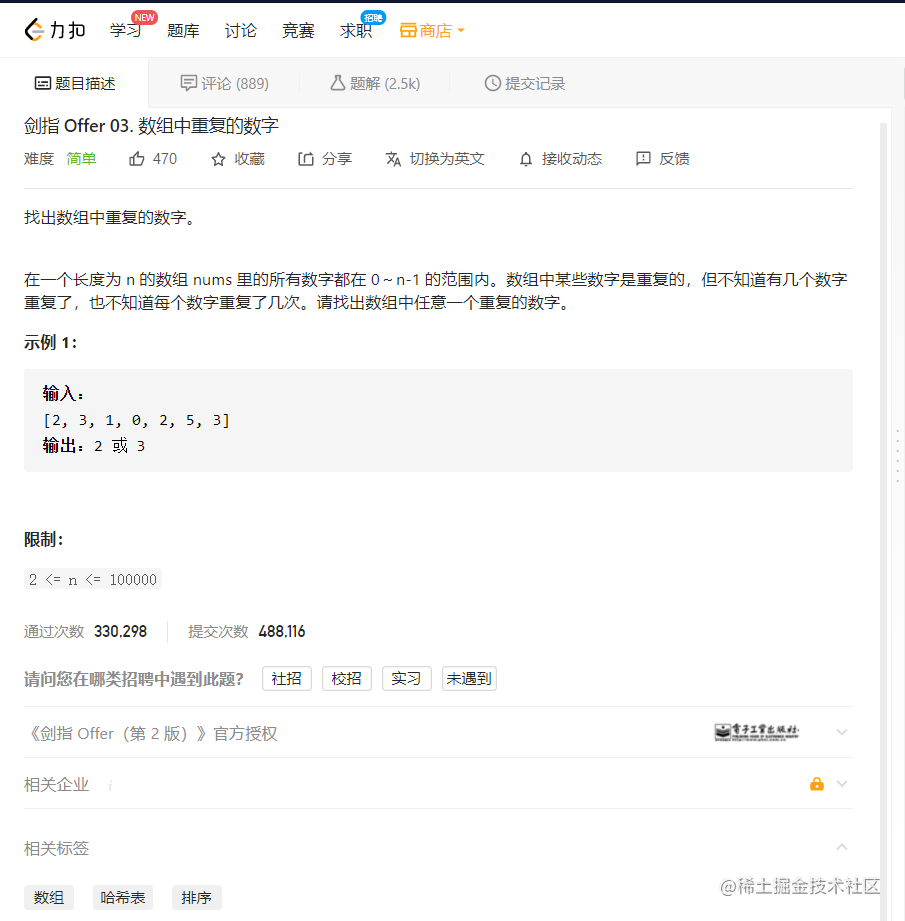## 2、哈希表

1. 初始化： 新建哈希表 map，记为 hash，第一个位置是数字，第二个位置是次数；
2. 遍历数组 nums 中的每个数字 nums[i] ：
1. 将 nums[i] 添加至 hash 中；
2. 当 nums[i] 在 hash 中的次数非 1，说明重复，直接返回 nums[i] ；
3. 返回 -1 ，找不到重复的数字，返回 -1 。
``````//面试题03.数组中重复的数字
//map存储
class Solution {
public:
int findRepeatNumber(vector<int>& nums) {
map<int, int> hash;
for(int i = 0; i < nums.size(); ++ i)
{
hash[nums[i]] ++ ;
if(hash[nums[i]] != 1) return nums[i];
}
return -1;
}
};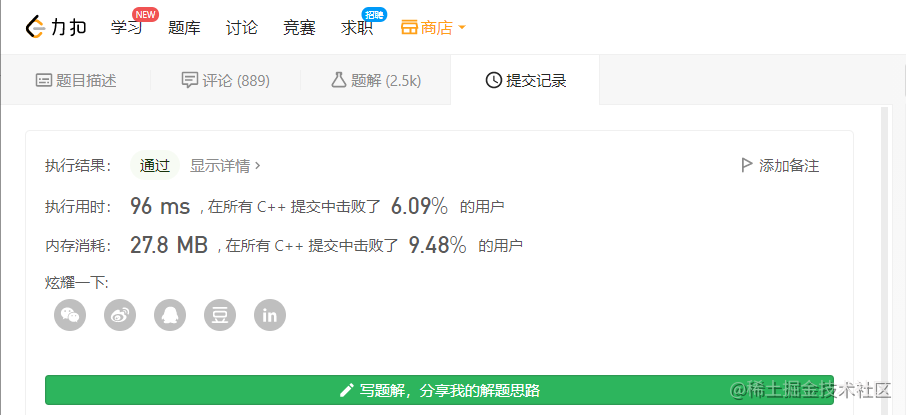``````//等效代码
class Solution {
public:
int findRepeatNumber(vector<int>& nums) {
//unordered_map<int, int> hash;
map<int, int> hash;
//for(int i = 0; i < nums.size(); ++ i)
for(int num : nums)
{
hash[num] ++ ;
if(hash[num] != 1) return num;
}
return -1;
}
};

## 3、原地置换

1. 遍历数组 nums 中的每个数字 nums[i] ：
1. 将 nums[i] == nums[nums[i]]，说明该数字与该数字索引的数字相同；
2. 当 nums[i] != nums[nums[i]]，可以交换使之符合 1.1 中情况；
2. 如果 nums[i] == i，说明交换有效果了，否则返回答案 nums[i] ；
3. 返回 -1 ，找不到重复的数字，返回 -1
``````//面试题03.数组中重复的数字
//标准做法
class Solution {
public:
int findRepeatNumber(vector<int>& nums) {
for(int i = 0; i < nums.size(); ++ i)
{
while(nums[i] != nums[nums[i]]) swap(nums[i], nums[nums[i]]);
if(nums[i] != i) return nums[i];
}
return -1;
}
};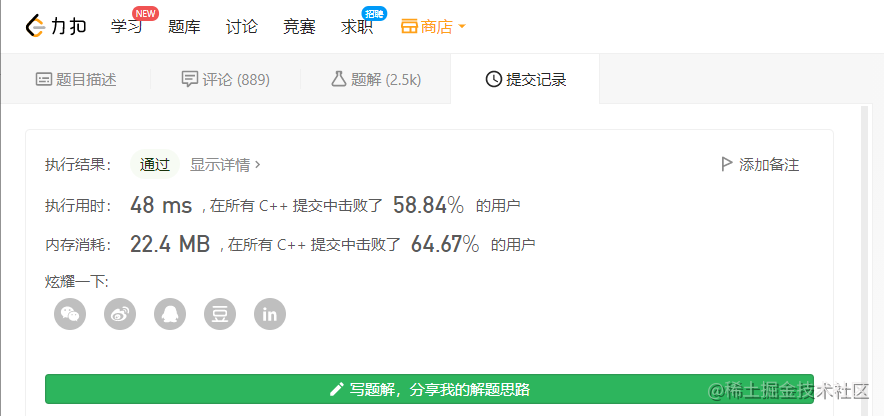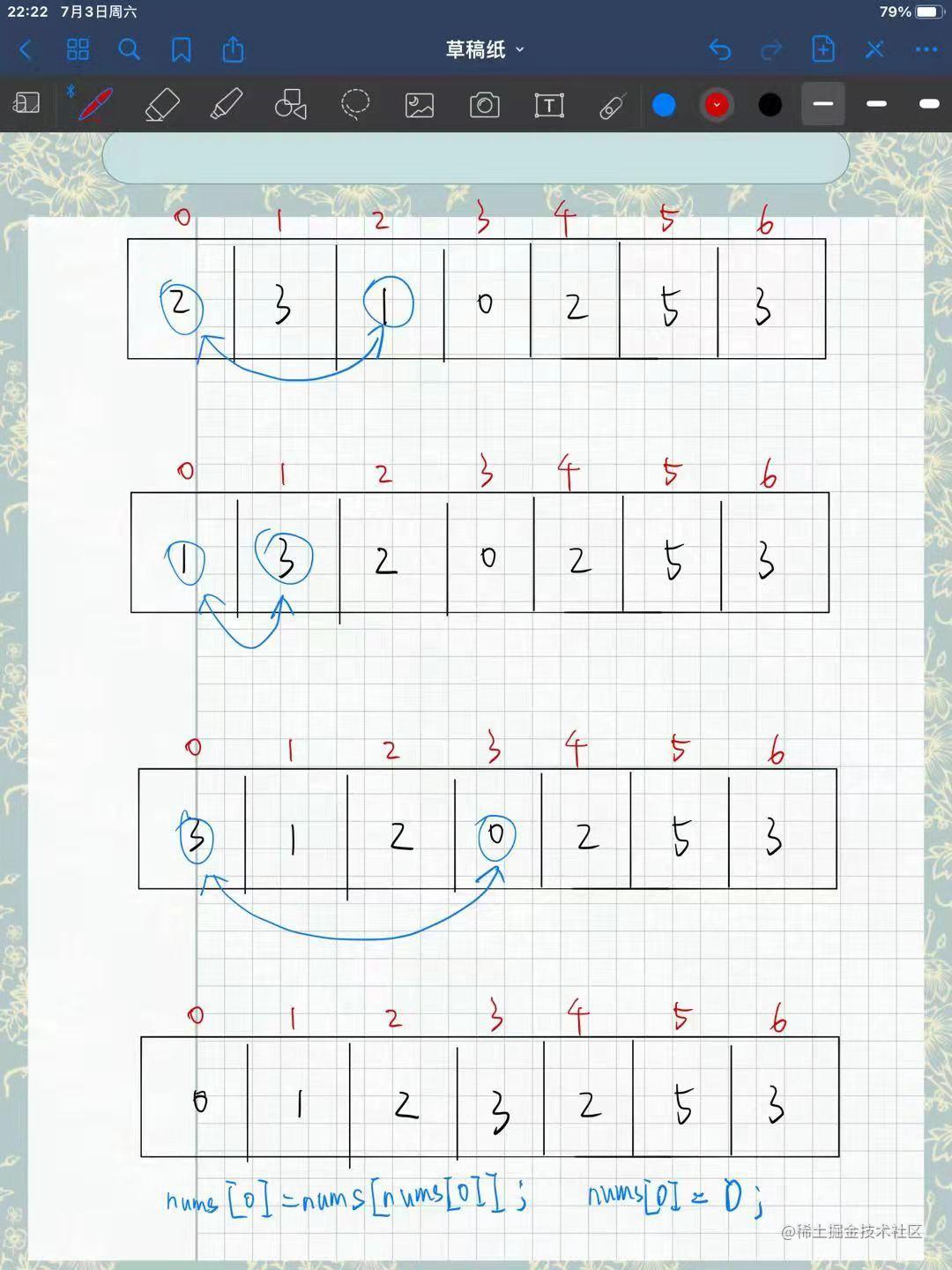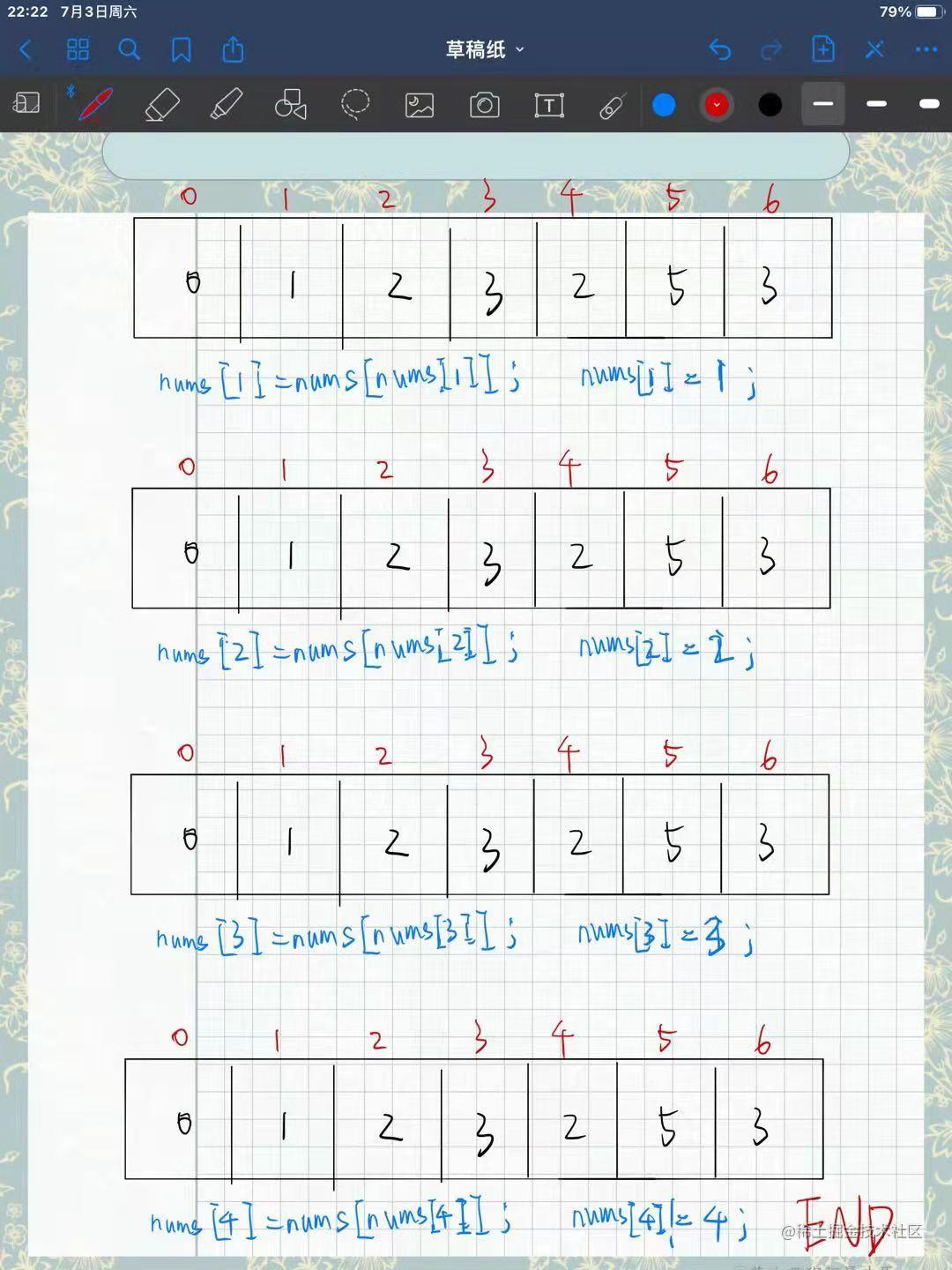## 4、复杂度

``````/*

*/

——————————————————————————————————————

—————————————————————————————————————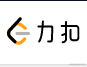leetcode-cn.com/u/tefuirnev…blog.nowcoder.net/wsguanxlmp.weixin.qq.com/s/bDwxwQfZy…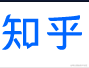www.zhihu.com/people/tefu…